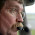## Sunday, September 13, 2015

### Arduino and Servos

This little project has been lying around waiting to be done for far to long, so I have resurrected it on a rainy Sunday and hope to make something useful out of it.

I have this little AZ/EL camera mount pair of servos that are quite stout.  They have external feedback potentiometers for the servo position, so even though they are geared down, they can be accurately positioned.

Coupling these up to an Arduino UNO is trivial.  I need one signal pin, power and ground.  Do not try to power servos from the USB power on the Arduino, use an external power supply.

The first task at hand was to decide on the range of pulse widths that would be allowed for the servo.  The defaults are 544 us to 2400 us.  The APIs decide that if you set a pulse width less than the minimum, then it must be an angle in degrees 0-359.  I didn't take the time to sort this out.  Instead, I decided arbitrarily that angle zero is 500 us. and everything is counterclockwise from there.

I placed a mark on the Azimuth gear right at the servo gear and through experimentation determined that a change of 768 microseconds would rotate the gear 360 degrees.

From there, it was simple to create a function that would convert an angle in degrees to the required microseconds pulse width to position the servo as desired.

// For the azimuth servo, angle is mapped 0-360 degrees to 500-128 us
int deg2usAZ(int angle)
{
return map(angle, 0, 360, 500, 1268);

}

Now in the case of the elevation servo, we have some limitations to the range of motion due to the physical characteristics of the assembly.  I decided first to figure out where level was and call this zero degrees of elevation.

Next I determined the setting for negative 45 degrees of elevation (pointing down).

And finally, I determined the setting for pointing straight up.  Now it was trivial to create a function to map degrees to these settings.

// For elevation servo, angle is mapped from -45-90 to 1112 to 2220 us
int deg2usEL(int angle)
{
return map(angle, -45, 90, 1112, 2220);

}

Now with the addition of a button, I have a simple test application that will postion to zero degrees azimuth and elevation when started and then move to 90 degrees elevation and 45 degrees of azimuth when the button is pressed..

// Servo test code

#include <Servo.h>

int button1 = 4; //button pin, connect to ground to move servo
int press1 = 0;

Servo servoEL;
Servo servoAZ;

void setup()
{
pinMode(button1, INPUT);
servoEL.attach(7, 500, 2400);
servoAZ.attach(9, 500, 2400);

servoEL.writeMicroseconds(deg2usEL(0));
servoAZ.writeMicroseconds(deg2usAZ(0));

digitalWrite(4, HIGH);
}

// For the azimuth servo, angle is mapped 0-360 degrees to 500-128 us
int deg2usAZ(int angle)
{
return map(angle, 0, 360, 500, 1268);
}

// For elevation servo, angle is mapped from -45-90 to 1112 to 2220 us
int deg2usEL(int angle)
{
return map(angle, -45, 90, 1112, 2220);
}

void loop()
{
if (press1 == LOW)
{
servoEL.writeMicroseconds(deg2usEL(90));
servoAZ.writeMicroseconds(deg2usAZ(45));
}
else
{
servoEL.writeMicroseconds(deg2usEL(0));
servoAZ.writeMicroseconds(deg2usAZ(0));
}

}

So, next I obtained the Arduino port of Plan13 which is a port of some very old basic code for determining a satellite location given the latitude/longitude/elevation of an observer, the Keplerian Elements for the satellite of interest and the date/time of interest. Keplerian Elements (or just Keps), named after Johann Kepler [1571-1630] are a set of seven numbers called satellite orbital elements that define an ellipse oriented about the earth and place the satellite on the ellipse at a particular time.

I have the Arduino port of Plan13 up and running and calculating azimuth and elevation values.  The precision is really insufficient on the Arduino to have very accurate calculations due to the fact that double precision floating point is required, but the Arduino defines double precision as a single precision value.  So the calculations do not have the precision they might otherwise have, but I find it is quite sufficient for simple experiments like this.

The remaining work is to provide a little more glue logic to have what amounts to a desktop pointer to the satellite as it passes overhead.

More to come.
.

1.2.3.